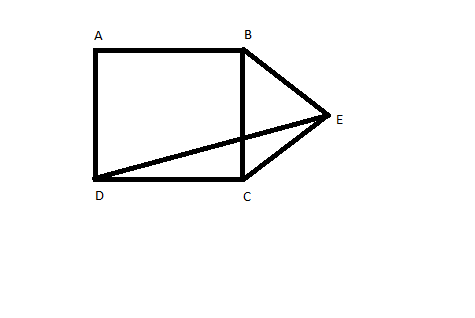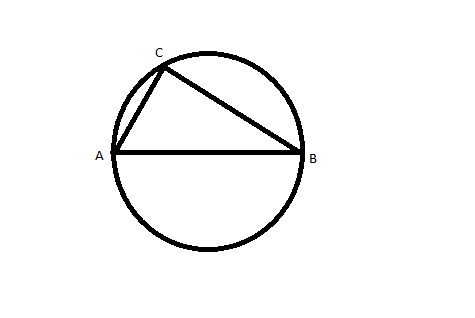# CAT 1996

Instructions

For the following questions answer them individually

Question 11

If ABCD is a square and BCE is an equilateral triangle, what is the measure of ∠DEC?Question 12

Instead of a metre scale, a cloth merchant uses a faulty 120 cm scale while buying, but uses a faulty 80 cm scale while selling the same cloth. If he offers a discount of 20%, what is his overall profit percentage?

Question 13

From a circular sheet of paper with a radius 20 cm, four circles of radius 5 cm each are cut out. What is the ratio of the uncut to the cut portion?

Question 14

A wooden box  of thickness 0.5 cm, length 21 cm, width 11 cm and height 6 cm is painted on the inside. The expenses of painting are Rs. 70. What is the rate of painting per square centimetres?

Instructions

Answer the questions based on the following information. A, S, M and D are functions of x and y, and they are defined as follows.

$$A(x, y)=x + y$$

$$S(x, y)=x-y$$

$$M(x, y)=xy$$

$$D(x,y)=\frac{x}{y}$$. $$y\neq0$$

Question 15

What is the value of $$M(M(A(M(x, y),S(y, x)),x),A(y, x))$$for $$x=2, y=3$$?

Question 16

What is the value of $$S[M(D(A(a, b), 2), D(A(a, b), 2)), M(D(S(a, b), 2), D(S(a, b), 2))]$$?

Instructions

For the following questions answer them individually

Question 17

The cost of diamond varies directly as the square of its weight. Once, this diamond broke into four pieces with weights in the ratio 1 : 2 : 3 : 4. When the pieces were sold, the merchant got Rs. 70,000 less. Find the original price of the diamond.

Question 18

If n is any odd number greater than 1, then $$n(n^2 - 1)$$ is

Question 19

The figure shows a circle of diameter AB and radius 6.5 cm. If chord CA is 5 cm long, find the area of triangle ABC.Instructions

Answer the questions based on the following information. A watch dealer incurs an expense of Rs. 150 for producing every watch. He also incurs an additional expenditure of Rs. 30,000, which is independent of the number of watches produced If he is able to sell a watch during the season, he sells it for Rs. 250. If he fails to do so, he has to sell each watch for Rs. 100.

Question 20

If he is able to sell only 1,200 out of 1,500 watches he has made in the season (and the rest 300 are sold out of season), then he has made a profit of

OR